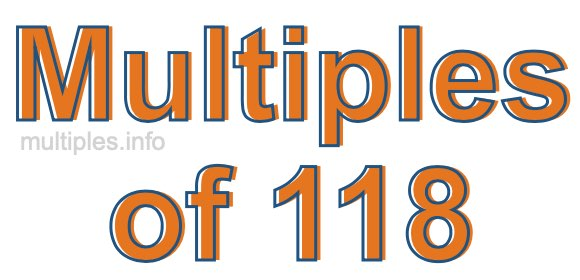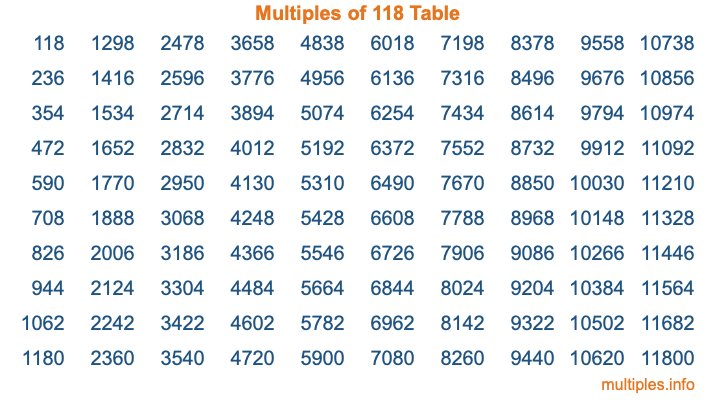Multiples of 118Welcome to the Multiples of 118 page. Here we will first teach you everything you will ever need to know about the multiples of 118, and then give you a study guide summary of everything we taught you to make sure you remember it all. Use this page to look up facts and learn information about the multiples of 118. This page will make you a multiples of one hundred eighteen expert!

Definition of Multiples of 118
Multiples of 118 are all the numbers that when divided by 118 equal an integer. Each of the multiples of 118 are called a multiple. A multiple of 118 is created by multiplying 118 by an integer.

Therefore, to create a list of multiples of 118, you start with 1 multiplied by 118, then 2 multiplied by 118, then 3 multiplied by 118, and so on for as long as you want. Thus, the list of the first five multiples of 118 is 118, 236, 354, 472, and 590. To see a larger list of multiples of 118, see the printable image of Multiples of 118 further down on this page. We also have a category where you can choose any nth multiple of 118.

Multiples of 118 Checker
The Multiples of 118 Checker below checks to see if any number of your choice is a multiple of 118. In other words, it checks to see if there is any number (integer) that when multiplied by 118 will equal your number. To do that, we divide your number by 118. If the the quotient is an integer, then your number is a multiple of 118.

Is  a multiple of 118?

Least Common Multiple of 118 and ...
A Least Common Multiple (LCM) is the lowest multiple that two or more numbers have in common. This is also called the smallest common multiple or lowest common multiple and is useful to know when you are adding our subtracting fractions. Enter one or more numbers below (118 is already entered) to find the LCM.

Check out our LCM Calculator if you need more details about the Least Common Multiple or if you need the LCM for different numbers for adding and subtraction fractions.

nth Multiple of 118
As we stated above, 118 is the first multiple of 118, 236 is the second multiple of 118, 354 is the third multiple of 118, and so on. Enter a number below to find the nth multiple of 118.

th multiple of 118

Multiples of 118 vs Factors of 118
118 is a multiple of 118 and a factor of 118, but that is where the similarities end. All postive multiples of 118 are 118 or greater than 118. All positive factors of 118 are 118 or less than 118.

Below is the beginning list of multiples of 118 and the factors of 118 so you can compare:

Multiples of 118: 118, 236, 354, 472, 590, etc.

Factors of 118: 1, 2, 59, 118

As you can see, the multiples of 118 are all the numbers that you can divide by 118 to get a whole number. The factors of 118, on the other hand, are all the whole numbers that you can multiply by another whole number to get 118.

It's also interesting to note that if a number (x) is a factor of 118, then 118 will also be a multiple of that number (x).

Multiples of 118 vs Divisors of 118
The divisors of 118 are all the integers that 118 can be divided by evenly. Below is a list of the divisors of 118.

Divisors of 118: 1, 2, 59, 118

The interesting thing to note here is that if you take any multiple of 118 and divide it by a divisor of 118, you will see that the quotient is an integer.

Multiples of 118 Table
Below is an image of the first 100 multiples of 118 in a table. The table is in chronological order, column by column. The first column has the first ten multiples of 118, the second column has the next ten multiples of 118, and so on.The Multiples of 118 Table is also referred to as the 118 Times Table or Times Table of 118. You are welcome to print out our table for your studies.

Negative Multiples of 118
Although not often discussed or needed in math, it is worth mentioning that you can make a list of negative multiples of 118 by multiplying 118 by -1, then by -2, then by -3, and so on, to get the following list of negative multiples of 118:

-118, -236, -354, -472, -590, etc.

Multiples of 118 Summary
Below is a summary of important Multiples of 118 facts that we have discussed on this page. To retain the knowledge on this page, we recommend that you read through the summary and explain to yourself or a study partner why they hold true.

There are an infinite number of multiples of 118.

A multiple of 118 divided by 118 will equal a whole number.

118 divided by a factor of 118 equals a divisor of 118.

The nth multiple of 118 is n times 118.

The largest factor of 118 is equal to the first positive multiple of 118.

118 is a multiple of every factor of 118.

118 is a multiple of 118.

A multiple of 118 divided by a divisor of 118 equals an integer.

118 divided by a divisor of 118 equals a factor of 118.

Any integer times 118 will equal a multiple of 118.

Multiples of a Number
Here you can get the multiples of another number, all with the same attention to detail as we did for multiples of 118 on this page.

Multiples of
Multiples of 119
Did you find our page about multiples of one hundred eighteen educational? Do you want more knowledge? Check out the multiples of the next number on our list!